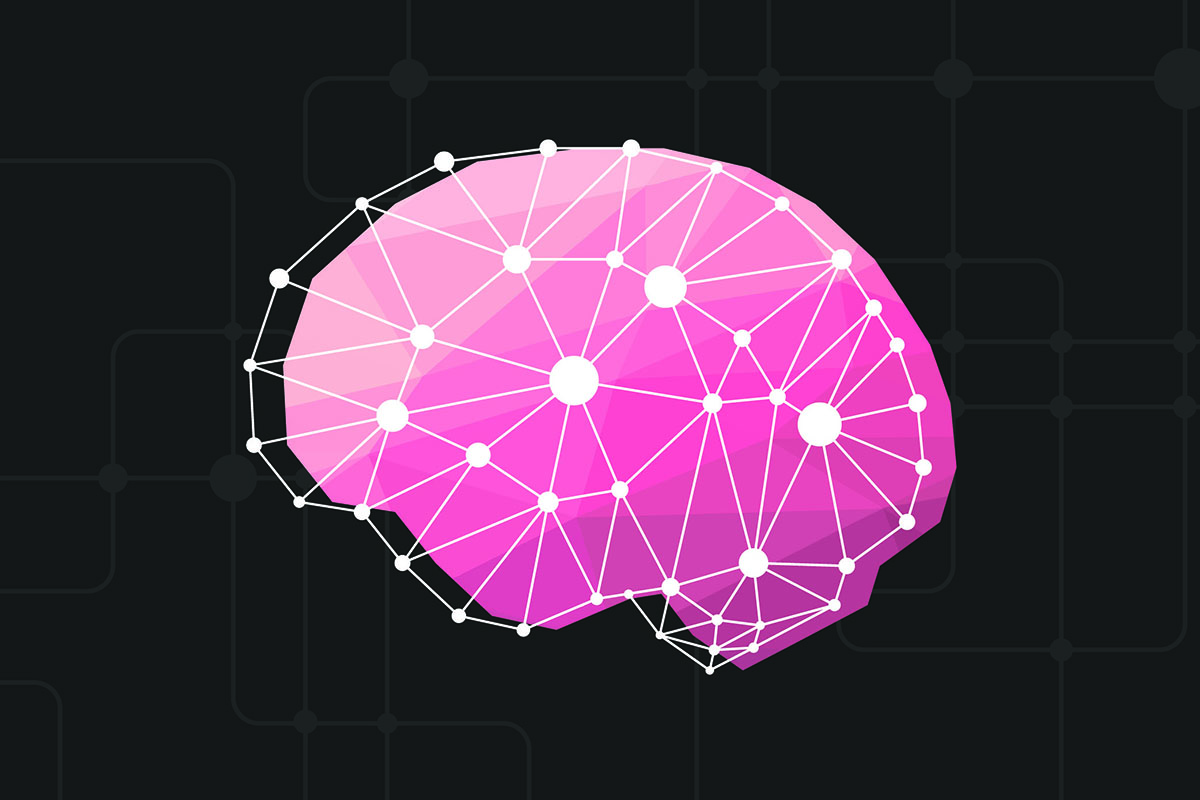##### Convolutional neural network

The convolutional neural network (CNN, or ConvNet) is an artificial neural network (ANN) class commonly used to analyze visual imagery in deep learning. CNNs, which provide …

Sept. 22, 2022 1 minuteThe convolutional neural network (CNN, or ConvNet) is an artificial neural network (ANN) class commonly used to analyze visual imagery in deep learning. CNNs, which provide translation-equivariant responses, are also known as Shift Invariant or Space Invariant Artificial Neural Networks (SIANN). Their shared-weight architecture is based on convolution kernels or filters that slide along input features. As a result of the downsampling operation, they apply to the input, and most convolutional neural networks are not invariant to translation. CNN's are used in image and video recognition, recommender systems, image classification, image segmentation, medical image analysis, natural language processing, brain-computer interfaces, and financial time series analysis.What is a convolutional neural network?

A convolutional neural network (CNN) processes pixel data in image recognition and processing. A convolutional neural network (CNN) processes pixel data in image recognition and processing. CNNs are artificial intelligence (AI) that use deep learning to perform productive and descriptive tasks, machine vision for image and video recognition, recommender systems, and natural language processing (NLP).How do convolutional neural networks work?

Convolutional neural networks perform better with image, speech, or audio inputs than other neural networks. They have three main classes of layers, which are:

• The convolutional layer
• The pooling layer
• Layers that are fully connected (FC)

Convolutional networks begin with the convolutional layer. Following convolutional layers, pooling or further convolutional layers can be added, but the fully-connected layer is the final layer. CNNs become more complex as they add layers, allowing them to identify more significant portions of the image. A more superficial feature, such as a color or an edge, is emphasized in the earliest layers. And with each successive layer of the CNN, the image data begins to recognize more significant object elements or shapes until it finally recognizes the intended object.

How do we calculate parameters for the convolutional neural network?

We must calculate each convolutional layer's size based on the input image's size. A CNN's output layer is calculated by dividing the input size by (image_size - (filter_size-1)). If the input image_size is (50,50) and the filter is (3,3), then (50-(3–1)) = 48.A convolutional neural network cascade for face detection?

Detecting faces, in reality, requires an advanced discriminative model that accurately distinguishes faces from backgrounds due to significant variations in pose, expression, and lighting. Consequently, effective models are often computationally prohibitive. To address these two challenges, we propose a cascade architecture built on convolutional neural networks (CNNs) that can discriminate while maintaining high performance. A CNN cascade operates at multiple resolutions, rejects the background regions quickly in low-resolution stages, and carefully evaluates a few challenging candidates in the high-resolution stage.Conclusion

The convolutional neural network is an artificial neural network (ANN) class commonly used to analyze visual imagery in deep learning. CNNs are artificial intelligence (AI) that use deep learning to perform productive and descriptive tasks, machine vision for image and video recognition, recommender systems, and natural language processing (NLP).

CNN's have three main layers: the convolutional layer, the pooling layer, and the fully connected (FC) layer. A CNN cascade operates at multiple resolutions and rejects the background regions quickly in low-resolution stages.